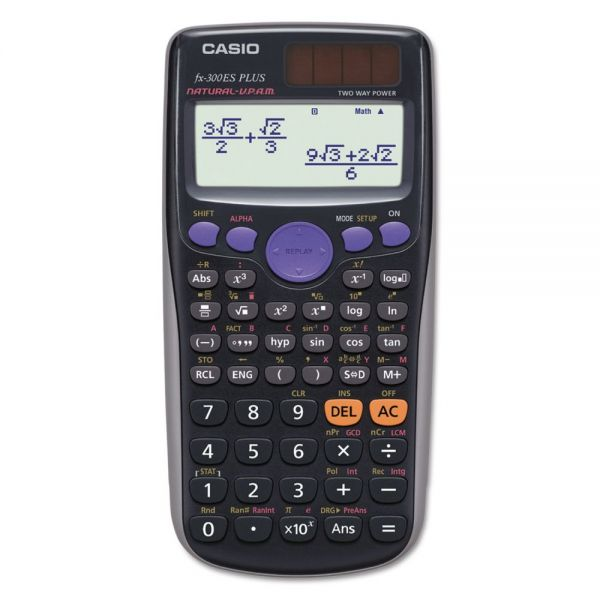# Casio FX-300ESPLUS Scientific Calculator, 10-Digit, Natural Textbook Display, LCD

Product Number: CSOFX300ESPLUS
• Intuitive functions.
• Improved math functionality.
• Natural textbook display shows formula and results exactly as they appear in the textbook.
• 252 built-in functions with easy menu.
More details
\$12.04
2-Day Shipping Estimated Delivery
Favorite
Compare
Request Quote

## Product Description

Casio FX-300ESPLUS Scientific Calculator, 10-Digit, Natural Textbook Display, LCD - Scientific calculator features natural textbook display and improved math functionality. 252 built-in functions with easy menu. Designed as the perfect choice for middle school through high school students learning General Math, Trigonometry, Statistics, Algebra I and II, Pre-Algebra, Geometry, Physics.

• Intuitive functions.
• Improved math functionality.
• Natural textbook display shows formula and results exactly as they appear in the textbook.
• 252 built-in functions with easy menu.Guides & Information

## Product Specifications

• +/- Switch Key
Yes
• Amortization
No
• Assembly Required
No
• Backspace Key
No
• Base Number Calculations
No
• Bond Calculations
No
• Brand Name
Casio
• Case
Slide-On Hard-Case
• Cash Flow Calculations
No
• Complex Number Calculations
No
• Confidence Interval Calculating
No
• Cost/Sell/Margin
No
• Country of Origin
CN
• Currency Exchange Function
No
• Date Calculations
No
• Decimal Function
Yes
• Depreciation Calculations
No
• Display Angle
Fixed
• Display Characters Height
4 mm
• Display Characters x Display Lines
10 x 2
• Display Notation
Natural Textbook Scientific
• Display Type(s)
LCD
• Display Window Resolution
LCD
• Double Zero Key
No
• Entry Logic
Natural V.P.A.M.
• Equation Editor
No
• Fraction Calculations
Yes
• Fraction/Decimal Conversions
Yes
• Global Product Type
Calculators-Scientific
• Grand Total Key
No
• Graphing Functions
No
• Higher Mathematical Functions
Algebra I & II General Math Geometry Physics Pre-Algebra Statistics Trigonometry
• Hyperbolic Functions
Yes
• Hypothesis Testing
No
• I/O Port
No
• Interest Rate Conversion
No
• Item Count Function
No
• Linear Regression
Yes
• Loan Calculation
No
• Logical (Boolean) Operations
No
• Markup/Down Key
No
• Matrices
No
• Memory
1 Independent 9 Constant
• Metric Conversion
No
• Number of Display Digits
10
• Percent Add-On/Discount
No
• Percent Key(s)
No
• Polar-Rectangular Conversion
No
• Post-Consumer Recycled Content Percent
0%
• Power Source(s)
Battery Solar
• Pre-Consumer Recycled Content Percent
0%
• Probability (Random Number)
Yes
• Programming Steps
No
• Quantity
1 each
• Recycled
No
• Replacement Batteries
LR44
• Simultaneous Equations
No
• Size
3 1/8 x 6 3/8
• Square Root Key
Yes
• Tax Calculation
Yes
• Time-Value-of-Money
No
• Time/Date
No
• Total Recycled Content Percent
0%
• Trig/Log Functions
Yes
• Variable Regression
Yes
• Variable Statistics
Yes
• Wall-Mountable
No

2.5 2 reviews